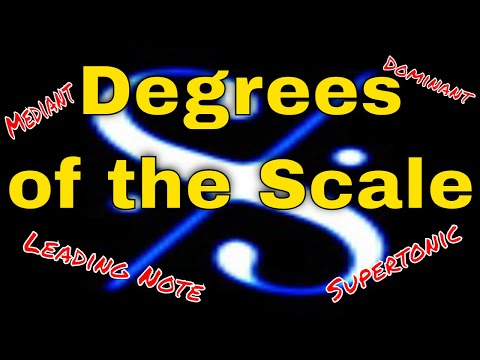## Search This Site### Lesson 4.7 - Technical Names for Degrees of the Scale

This requirement is simply a question of learning seven words corresponding to the seven degrees of the scale - and you already know the first one!

In Trinity College Theory they are introduced one or two at a time from  Grades 1-6 whereas in ABRSM Music Theory, they are all introduced together at Grade 4.

1st degree of the scale = tonic
2nd degree of the scale = supertonic
3rd degree of the scale = mediant
4th degree of the scale = subdominant
5th degree of the scale = dominant
6th degree of the scale = submediant
7th degree of the scale = leading note
8th degree of the scale = tonic (same as 1st)

There is a pattern to observe that might help you remember some of these. The prefix "sub" means below. So if the dominant is the 5th note up from the tonic, then the subdominant is the 5th note  down  from the tonic. The same is true for mediant (3rd note up) and submediant (3rd note down)